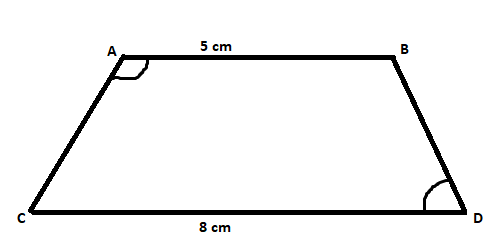Home/Class 8/Maths/

$$ABCD$$ is a quadrilateral in which $$AB=5cm,CD=8cm$$ and the sum of angle$$A$$and angle $$D$$ is $$180^{\circ }$$. What is the name of this quadrilateral?（ ）
A. Parallelogram
B. Trapezium
C. Rhombus
D. Can not be determinedSpeed
00:00
03:55## QuestionMathsClass 8

$$ABCD$$ is a quadrilateral in which $$AB=5cm,CD=8cm$$ and the sum of angle$$A$$and angle $$D$$ is $$180^{\circ }$$. What is the name of this quadrilateral?（ ）
A. Parallelogram
B. Trapezium
C. Rhombus
D. Can not be determined

B
4.64.6## Solutionwe have given that $$ABCD$$ is a quadrilateral as we can see in the figure above the sides $$AB=5\ cm$$ and side $$CD = 8\ cm$$.
$$\angle A +\angle D = 180^{\circ}$$ (given)
If a quadrilateral have sum of supplementary angle as $$180^{\circ}$$ and the opposite sides are unequal(given) then the quadrilateral is a Trapezium.
Hence, Quadrilateral $$ABCD$$ is a Trapezium.